# Identification of Vortex Structures in Flow Field Images Based on Convolutional Neural Network and Dynamic Mode Decomposition

Identification of Vortex Structures in Flow Field Images Based on Convolutional Neural Network and Dynamic Mode Decomposition
School of Aeronautics and Astronautics, Zhejiang University, Hangzhou 310027, China

School of Aeronautics and Astronautics, Zhejiang University, Hangzhou 310027, China

Corresponding Author Email:
ye_zhixian@zju.edu.cn
Page:
501-506
|
DOI:
https://doi.org/10.18280/ts.360604
2 April 2019
|
Accepted:
8 August 2019
|
Published:
29 December 2019
| Citation

OPEN ACCESS

Abstract:

The generation and evolution of turbulence are affected by the way vortex structures emerge and interact with each other. The quick identification of vortex in flow field will offer a simple and easy way to explore the mechanism of turbulent flow. This paper combines convolutional neural network (CNN) and dynamic mode decomposition (DMD) into a novel vortex structure identification method, based on the flow field images obtained by simulation of the flow around circular cylinder and experiments of synthetic jet actuators. DMD was adopted to modally decompose the instantaneous image sequence of the flow field over a period and the first primary mode was added to the limit loss function of CNN vortex identification. Then a novel CNN topology was designed and trained by flow field image sequence. The proposed neural network managed to recognize 96% of the vortex structures correctly in the flow field images of the flow around circular cylinder and the synthetic jet flow. The research results provide a new way to identify vortex structures.

Keywords:

image processing, vortex identification, convolutional neural network (CNN), dynamic mode decomposition (DMD)

1. Introduction

Vortex is a common form of fluid motion in the flow field. And turbulence contains multiple vortex structures of different scales and intensities. In fact, the generation and evolution of turbulence are directly affected by the way vortex structures emerge and interact with each other . Therefore, the accurate identification of vortex structures in the flow field is of great significance for understanding the turbulent flow mechanism and controlling turbulent flow [2, 3]. Some common methods for vortex identification are the Q criterions, $\lambda_{2}, \Delta,$ and $\lambda_{c i}$, and all these criterions need to process the velocity information of the flow field, and select the proper thresholds based on Cauchy-Stokes decomposition and the eigenvalues of velocity gradient tensor V [4-8].

In engineering applications, the direct and convenient way to obtain the flow field information is capturing the images of the instantaneous flow field, including experimental measurements and numerical simulations. But high computing load and time cost will be incurred, if the flow field velocity is calculated based on the image information with positioning the vortex by physical equations. Directly processing the images of the instantaneous flow field and pinpoint the vortex structures in the images will simplify and speed up the vortex identification.

The convolutional neural network (CNN) provides a new solution to vortex identification based on the flow field image. The CNN is the most widely used deep learning technique. It has been applied extensively in target recognition, image classification and object detection. With the aid of the CNN, it is possible to position the vortex structures while acquiring the flow field images at the same time.

LeCun et al.  proposed the LeNet, the prototype of modern CNN, for 2D image recognition. As shown in Figure 1, the LeNet consists of 2 convolutional layers, 2 pooling layers, and 1 fully-connected layer. The image features are extracted in convolutional and pooling layers; the data of the original image are mapped to the feature dimension, and then classified in the fully-connected layer; the feature dimension is mapped to the sample labels. The LeNet has been successfully applied to different image recognition tasks, and hailed as a universal image recognition neural network.

## 1.png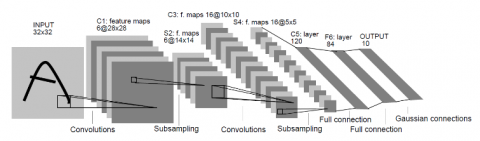Figure 1. Structure of the LeNet 

Based on the LeNet, Krizhevsky et al.  put forward the AlexNet, which has 5 convolutional layers and 3 fully-connected layers, more than those in the LeNet. The AlexNet adopts distributed computing to speed up network training and improve the efficiency of image recognition. It is the first neural network that made a breakthrough in the ImageNet Large Scale Visual Recognition Competition (ILSVRC). The VGG model, designed by Simonyan  of Oxford University, is deeper than the AlexNet, and enhances the performance of the CNN in image classification. Szegedy  from Google presented the InceptionNet, which integrates multiscale model structures by increasing the number of image feature extraction layers. Because it is easy to learn the residuals between input and output using the convolutional layer, He Kaiming et al.  developed the ResNet to curb the reduction of image classification accuracy caused by the growing network depth.

The common CNNs like the VGG and ResNet can identify the class of each image, but only in a rough manner. By contrast, the fully convolutional network (FCN)  can classify images at the pixel level. The FCN accepts input images of any size, and predicts each pixel in the images, without sacrificing their spatial information. The classification is performed pixel by pixel, solving the semantic segmentation problem. For example, the U-Net has a very high recognition rate on medical images. This horizontally crossed CNN can identify tumor features on medical computed tomography (CT) images [15, 16]. DeepLab , a deep CNN, is widely used in autonomous driving, thanks to its high recognition rate of pedestrians and vehicles in road images collected by onboard cameras.

To identify vortex in the flow field, Brendan Colvert et al.  correctly classified the different fluid behaviors in the flow field by neural network, according to the physical equations of vortex calculation. Lguensat et al.  developed a deep neural network with EddyNet structure, and constructed a pixel-level classification system for ocean eddies. To improve the pixel-level recognition rate of flow field images, the key is to make full use of the effective information in the flow field and add it to the structure of the CNN. The dynamic mode decomposition (DMD) can perform modal decomposition of continuous images, treating them as input snapshots, and extract features from the flow field images obtained from experiments or calculations, providing suitable data sources for the CNN. Proposed by Schmid  in 2008, the DMD is suitable for numerical analysis of experimental data on flow field. By this method, the spatiotemporal coherent information of complex flow images can be decomposed into several main modalities, and effective information can be extracted from high-dimensional data, laying the basis for short-term prediction and control.

In this paper, the CNN and the DMD are combined to identify and predict the vortex structures in the flow field images obtained through experiments and numerical simulations. Firstly, the DMD was adopted to modally decompose the instantaneous image sequence of the flow field over a period, revealing the first primary mode. The modes with amplitude greater than a fixed value were defined as the effective area of the vortex, and used as the limit loss function of vortex classification. Next, a novel topology like the U-Net was designed, in which each convolutional layer at the bottom is up-sampled to the corresponding pooling layer and added up layer by layer. The flow field image sequence was then imported for the identification of vortex structures. The proposed neural network was trained by hundreds of thousands of experimental data. The trained network correctly recognized 96% of the vortex in the flow field images.

2. Image Acquisition and Processing

2.1 Acquisition of flow field images

As shown in Figure 2, the flow field data were obtained by computing the flow around a circular cylinder in numerical simulation software. The velocity and vorticity of the flow field were computed based on the following parameters: incoming flow velocity, 1m/s; cylinder diameter, 10mm; actual grid size, 200mm×100mm; kinematic viscosity of gas, 0.15. The grid density was increase in local areas.

## 2.pngFigure 2. The calculation of the flow around a circular cylinder

To acquire flow field images through experiments, a synthetic jet actuator with a slot-shaped outlet was designed, and used to obtain the images of the jet flow field. As shown in Figure 3, the actuator is composed of a loudspeaker, a cavity and an outlet cover. Once the excitation signal is imported, the loudspeaker cone moves up and down reciprocally, compressing and expanding the gas in the cavity. In this way, the gas is pushed out and pull in periodically via the outlet, forming a synthetic jet.

In addition, a time-resolved particle image velocimetry (TR-PIV) system (Figure 4) was set up to illuminate the flow field near the outlet with a laser. The instantaneous flow field near the outlet was captured by a high-speed camera.

## 3.png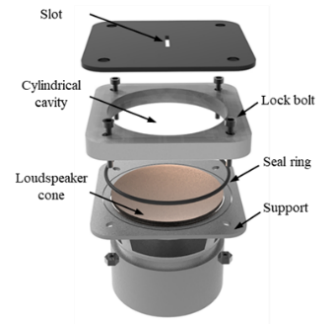Figure 3. The synthetic jet actuator

## 4.png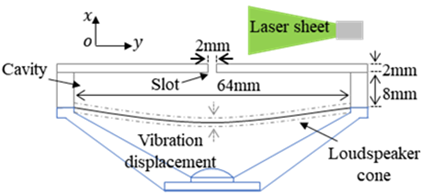Figure 4. The TR-PIV system

To verify its effectiveness, our neural network model was applied to process the images of three different flow fields, namely, the flow field of the flow around a circular cylinder, the flow field of the synthetic jet flow excited by low voltage, and flow field of the synthetic jet flow excited by high voltage.

2.2 The DMD

The flow field information obtained by experiments and numerical simulations was inputted as image snapshots. The Koopman operator A was introduced to map each snapshot from $v^{i} \operatorname{to} v^{(i+1)}$:

$v^{(i+1)}=A v^{(i)}$

Then, $V_{1}^{N}$ can be constructed as a Krylov sequence:

$V_{1}^{N}=\left[\begin{array}{cccc}{v^{(1)}} & {A v^{(1)}} & {A^{2} v^{(1)}} & {\cdots} & {A^{N-1} v^{(1)}}\end{array}\right]$

According to the assumption of linear mapping, Koopman operator A can reflect the dynamic features of how the snapshot evolves over time. Hence, the solution of matrix A is the key to the DMD. In general, the matrix is solved by dimensionality reduction.

To perform reduced order analysis of the snapshot sequence, the $V_{1}^{N}$ can be characterized by several DMD modes, which is far fewer than N-1. If D+1 DMD modes are selected, then the order reduction can be expressed as:

$V_{1}^{N}=\left[v^{(1)} v^{(2)} \ldots v^{(N)}\right]=\left[\begin{array}{cc}{\psi_{0}} & {\psi_{1}} & {\cdots \psi_{T_{D}}}\end{array}\right]\left[\begin{array}{cccc}{\alpha_{0}} & {} & {} & {} \\ {} & {\alpha_{1}} & {} & {} \\ {} & {} & {\ddots} & {} \\ {} & {} & {} & {\alpha_{T_{D}}}\end{array}\right]\left[\begin{array}{cccc}{1} & {\mu_{0}} & {\cdots} & {\mu_{0}^{N-1}} \\ {1} & {\mu_{1}} & {\cdots} & {\mu_{1}^{N-1}} \\ {\vdots} & {\vdots} & {\ddots} & {\vdots} \\ {1} & {\mu_{T_{D}}} & {\cdots} & {\mu_{T_{D}}^{N-1}}\end{array}\right]$

2.3 CNN structure

In the conventional CNN, each convolutional layer is connected to several fully-connected layers. The feature map produced by the convolutional layer is mapped to a fixed-length feature vector, which applies to image-level classification and recognition. The conventional topology is not suitable for vortex identification in flow field images, because this task requires the classification of each pixel. To solve the problem, this paper adopts the topology of the FCN (Figure 5). The FCN accepts input images of any size. The feature map of the last convolutional layer is up-sampled on a deconvolutional layer, and thus restored to the size of the input image. Then, the restored image was expanded into a column, making every pixel predictable.

Of course, it is impossible to classify each pixel accurately by the up-sampling of the feature map of the last convolutional layer alone. This calls for integration based on skip layers. Due to the rapid structural changes in real flow field, there are various sizes of vortex structures in the flow field images. To fully utilize its parameters, the last convolutional layer, which involves numerous small-scale pixel features, was connected to the up-sampling layer.

## 5.png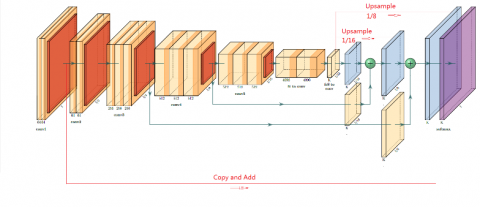Figure 5. The structure of the FCN

3. Results and Analysis

3.1 Extraction of image features

The DMD was employed to extract the information of vortex features in flow field images. The simulated flow around a circular cylinder (Figure 6) was decomposed by the DMD, revealing the relationship between vorticity and the first primary mode. Two types of data were obtained through simulation: the data on velocity field, and the pixel data of the images. Both types of data can be converted into matrices. However, the two types of data cannot be unified into a matrix, because of their difference in scale and the stored physical information. The ultimate purpose of the DMD is to modally decompose the images of the flow field and input the results into the neural network as the feature information of the flow field. Therefore, it is necessary to verify the rationality of the DMD modal decomposition of the data from the images, with the DMD decomposition of velocity field data as the reference.

## 6a.png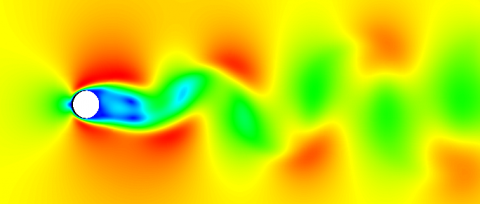(a) Velocity cloud map

## 6b.png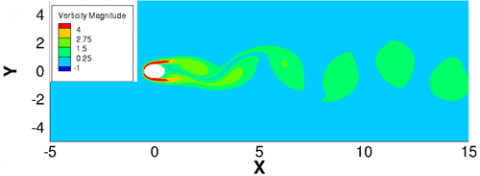(b) Vorticity cloud map

Figure 6. Simulated flow around a circular cylinder

To perform DMD modal decomposition of the flow field, a continuous sequence of flow field should be provided with a fixed time interval. Here, the flow around a circular cylinder was sampled at every 500μs, and inputted as snapshots. The first primary modes of the velocity field and the image data are displayed in Figure 7 below.

## 7a.png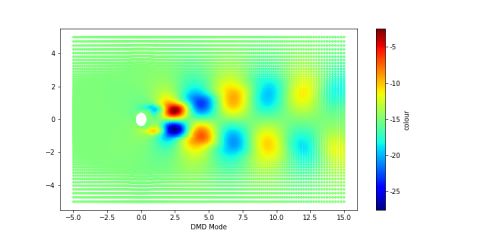(a) The first primary mode of the velocity field data

## 6b.png(b) The first primary mode of the image data

Figure 7. Modes of the flow around a circular cylinder obtained by the DMD

As shown in Figure 7, the first primary mode of the image data was more dispersed and less concentrated than that of the velocity field data. The difference arises from the processing of each pixel in the image. The pixel-by-pixel processing is equivalent to mean filtering and translation of flow field velocity, which induces a certain error in principal component decomposition. However, the two subfigures of Figure 7 only have a slight difference in overall shape and relative position, indicating that the loss function thresholds of the CNN can be selected suitably. During the numerical simulation of the flow around a circular cylinder, the flow field velocity images decomposed by the DMD can be applied in the CNN calculation.

The instantaneous velocity field, vorticity field, PIV and DMD primary mode of the synthetic jet flow field under high/low voltage excitation are displayed in Figures 8 and 9 below.

## 8a.png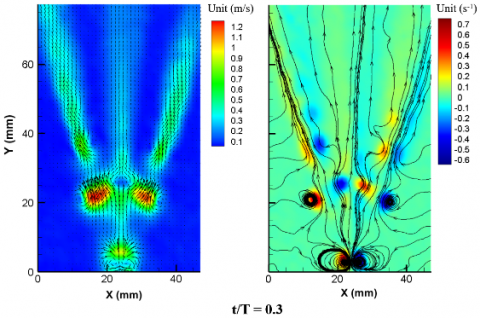(a) Velocity cloud map              (b) Vorticity cloud map

## 8c.png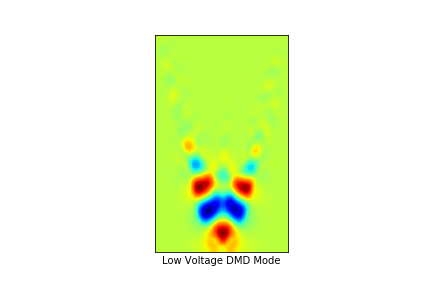(c) DMD primary mode

Figure 8. Flow field of the synthetic jet flow excited by low voltage

## 9a.png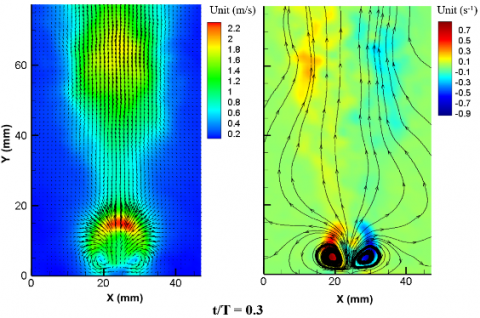(a) Velocity cloud map                   (b) Vorticity cloud map

## 9c.png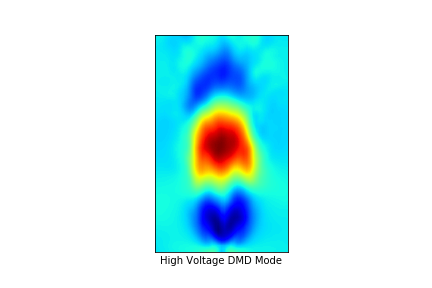(c)DMD primary mode

Figure 9. Flow field of the synthetic jet flow excited by high voltage

3.2 Identification results of vortex structures

As inputs to the neural network, the three different flow fields all have a certain periodicity, exhibiting obvious vortex structures. The information about vortex positions were extracted by the DMD from the flow field images. Through comparison, it is found that the first primary mode obtained by the DMD has a certain correlation with the vortex structures in the flow field: the relatively large points in the vorticity map all appeared near the dark areas, i.e. the maximum and minimum ranges of the amplitude of the primary mode. As a result, the vortex does not appear randomly the flow field. This is very effective information for pixel-level vortex identification. Taking this condition as a loss function of the CNN, the vortex structures in flow field images can be identified more accurately by the neural network.

During the training of the neural network, the size of each input image is 224×224×3, and the smallest convolutional layer is of the size 7×7×512. The convolutional layers are denoted as $F_{i},$ with $F_{6}$ being the smallest one. The deconvolutional layers (up-sampling layers) are denoted as $U_{i}$ from the left to right. The up-sampling is like the magnification of pixels. Because U1 was sampled from F6, the size of U2 can be expressed as U2=(F4/2+U1)×2+F6×4. U3 was formed in the same way as U2. The final layer was added with F1. In this way, the network structure covers the information of all scales, and the network acquires the ability of global perception.

To reduce errors and enhance the accuracy of image recognition, the first primary mode obtained by the DMD was linked with the vortex structure of the flow field, which further improves the loss function in the network. Specifically, any mode that deviates from the extreme value by less than 0.2 is denoted as M, the nonzero value of the vortex as W, and the M nearest to W as NearM. Let $\mathrm{P}_{\mathrm{X}}, \mathrm{P}_{\mathrm{Y}}$ be the pixel with the coordinates of x, y. Then, the effective distance of the vortex can be defined as:

$\mathrm{d}=\min \left(| W_{P x}-\text {NearM}_{p x}|,| W_{P y}-N e a r M_{p y} |\right)$

The image recognition error increases with the distance from the predicted vortex pixel to the NearM. The classification of vortex pixels is a binary classification problem. Here, the distance loss is combined with binary classification to suppress the false positive rate. The loss function can be defined as:

Loss $=-\sum_{i}\left[t_{i} \log \left(y_{i}\right)+\left(1-t_{i}\right) \log \left(1-y_{i}\right)\right]+\alpha\left(e^{d}\right.$$-1)$

## 10a.png(a) Vorticity map of the flow around a circular cylinder

## 10b.png(b) Vortex structures of the flow around a circular cylinder identified by the neural network

## 10c.png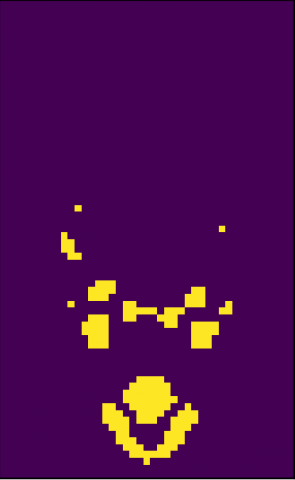(c) Vorticity map of the synth etic jet flow excited by low voltage

## 10d.png(d) Vortex structures of the synthetic jet flow excited by low voltage identified by the neural network

## 10e.png(e) Vorticity map of the synthetic jet flow excited by high voltage

## 10f.png(f)Vortex structures of the synthetic jet flow excited by high voltage identified by the neural network

Figure 10. Vorticity images and vortex identification results

The neural network was trained with the data of flow field images. Then, the images that have not been used as training samples were inputted to the network for vortex identification. The recognition results are shown in Figure 10. Compared with the vortex positions obtained by mathematical formulas, the vortex identified by the neural network in flow field images are accurate. The CNN did not suffer from over fitting, and recognized 96% of vortex positions correctly.

## 11a.png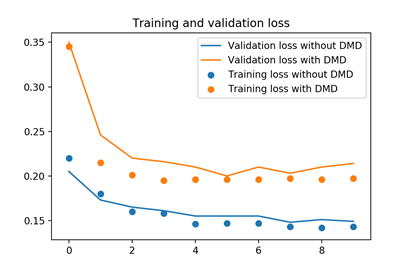(a) Concerning the flow around a circular cylinder

## 12.png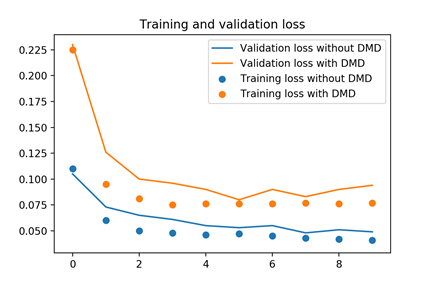(b) Concerning the synthetic jet flow excited by low voltage

Figure 11. Vortex identification errors and loss function values before and after the DMD

Before and after the DMD, the training and verification losses of the neural network concerning the flow around a circular cylinder and the synthetic jet flow excited by low voltage are compared in Figure 11. It can be seen that the CNN had a small error in training set, which is slightly greater than that in verification set. The errors in training and verification sets both decreased after the DMD was performed on the flow field image, and the DMD primary mode was introduced as part of the loss function. This means the DMD can clearly suppress errors.

4. Conclusions

Based on the CNN and DMD method, this paper proposes a novel neural network to identify the vortex structures in the flow field images around a circular cylinder and near the synthetic jet actuator. DMD was adopted to modally decompose the instantaneous image sequence of the flow field over a period. The first primary mode obtained was added to the limit loss function of the CNN network. Then the CNN topology was designed and trained by flow field image sequence. The proposed neural network managed to recognize 96% of the vortex structures in the flow field images.

References

 Pullin, D.I., Saffman, P.G. (1998). Vortex dynamics in turbulence. Annual Review of Fluid Mechanics, 30(1): 31-51. https://doi.org/10.1146/annurev.fluid.30.1.31

 Liu, C., Yan, Y., Lu, P. (2014). Physics of turbulence generation and sustenance in a boundary layer. Computers & Fluids, 102: 353-384. https://doi.org/10.1016/j.compfluid.2014.06.032

 Wallace, J.M. (2012). Highlights from 50 years of turbulent boundary layer research. Journal of Turbulence, (13): N53. https://doi.org/10.1080/14685248.2012.738907

 Robinson, S.K. (1991). Coherent motions in the turbulent boundary layer. Annual Review of Fluid Mechanics, 23(1): 601-639. https://doi.org/10.1146/annurev.fl.23.010191.003125

 Hunt, J.C.R., Wray, A.A., Moin, P. (1988). Eddies, streams, and convergence zones in turbulent flows. 89N24555, 19890015184.

 Jeong, J., Hussain, F. (1995). On the identification of a vortex. Journal of Fluid Mechanics, 285: 69-94. https://doi.org/10.1017/S0022112095000462

 Chong, M.S., Perry, A.E., Cantwell, B.J. (1990). A general classification of three-dimensional flow fields. Physics of Fluids A: Fluid Dynamics, 2(5): 765-777. https://doi.org/10.1063/1.857730

 Zhou, J., Adrian, R.J., Balachandar, S., Kendall, T.M. (1999). Mechanisms for generating coherent packets of hairpin vortices in channel flow. Journal of Fluid Mechanics, 387: 353-396. https://doi.org/10.1017/S002211209900467X

 LeCun, Y., Bottou, L., Bengio, Y., Haffner P. (1998). Gradient-based learning applied to document recognition. Proceedings of the IEEE, 86(11): 2278-2324. https://doi.org/10.1109/5.726791

 Krizhevsky, A., Sutskever, I., Hinton, G.E. (2012). Imagenet classification with deep convolutional neural networks. Advances in Neural Information Processing Systems, 25(2): 1097-1105. https://doi.org/10.1145/3065386

 Simonyan, K., Zisserman, A. (2014). Very deep convolutional networks for large-scale image recognition. arXiv preprint arXiv: 1409-1556.

 Szegedy, C., Liu, W., Jia, Y.Q., Sermanet, P., Reed, S., Anguelov, D., Erhan, D., Vanhoucke, V., Rabinovich, A. (2015). Going deeper with convolutions. Proceedings of the IEEE Conference on Computer Vision and Pattern Recognition, pp. 1-9. https://doi.org/10.1109/CVPR.2015.7298594

 He, K., Zhang, X., Ren, S., Sun, J. (2016). Deep residual learning for image recognition. Proceedings of the IEEE Conference on Computer Vision and Pattern Recognition, pp. 770-778. https://doi.org/10.1109/CVPR.2016.90

 Long, J., Shelhamer, E., Darrell, T. (2015). Fully convolutional networks for semantic segmentation. Proceedings of the IEEE Conference on Computer Vision and Pattern Recognition, pp. 3431-3440.https://doi.org/10.1109/CVPR.2015.7298965

 Kuruvilla, J., Gunavathi, K. (2014). Lung cancer classification using neural networks for CT images. Computer Methods and Programs in Biomedicine, 113(1): 202-209. https://doi.org/10.1016/j.cmpb.2013.10.011

 Jiang, J., Hu, Y.C., Tyagi, N., Zhang, P.P., Rimner, A., Mageras, G.S., Deasy, J.O., Veeraraghavan, H. (2018). Tumor-aware, adversarial domain adaptation from CT to MRI for lung cancer segmentation. International Conference on Medical Image Computing and Computer-Assisted Intervention. Springer, Cham, pp. 777-785. https://doi.org/10.1007/978-3-030-00934-2_86

 Chen, L.C., Papandreou, G., Kokkinos, I., Murphy, K., Yuille, A.L. (2017). Deeplab: Semantic image segmentation with deep convolutional nets, atrous convolution, and fully connected CRFS. IEEE Transactions on Pattern Analysis and Machine Intelligence, 40(4): 834-848. https://doi.org/10.1109/TPAMI.2017.2699184

 Colvert, B., Alsalman, M., Kanso, E. (2018). Classifying vortex wakes using neural networks. Bioinspiration & Biomimetics, 13(2): 025003. https://doi.org/10.1088/1748-3190/aaa787

 Lguensat, R., Sun, M., Fablet, R., Mason, E. (2018). EddyNet: A deep neural network for pixel-wise classification of oceanic eddies. IGARSS 2018-2018 IEEE International Geoscience and Remote Sensing Symposium. IEEE, pp. 1764-1767. https://doi.org/10.1109/IGARSS.2018.8518411

 Schmid, P.J. (2010). Dynamic mode decomposition of numerical and experimental data. Journal of Fluid Mechanics, 656: 5-28. https://doi.org/10.1017/S0022112010001217.